DIFFERENTIATION USING THE PRODUCT RULE

The following problems require the use of the product rule. In the following discussion and solutions the derivative of a function h(x) will be denoted byor h'(x) . The product rule is a formal rule for differentiating problems where one function is multiplied by another. The rule follows from the limit definition of derivative and is given by.

Remember the rule in the following way. Each time, differentiate a different function in the product and add the two terms together. In the list of problems which follows, most problems are average and a few are somewhat challenging. In most cases, final answers to the following problems are given in the most simplified form.

• PROBLEM 1 : Differentiate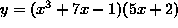.

• PROBLEM 2 : Differentiate.

• PROBLEM 3 : Differentiate.

• PROBLEM 4 : Differentiate.

• PROBLEM 5 : Differentiate.

• PROBLEM 6 : Differentiate.

The following problems require use of the chain rule.

• PROBLEM 7 : Differentiate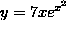.

• PROBLEM 8 : Differentiate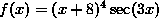.

• PROBLEM 9 : Differentiate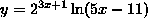.

• PROBLEM 10 : Differentiate.

• PROBLEM 11 : Differentiate.

• PROBLEM 12 : Differentiate.

• PROBLEM 13 : Consider the function. For what values of x is f'(x) = 0 ?

• PROBLEM 14 : Consider the function. For what values of x is f'(x) = 0 ?

• PROBLEM 15 : Consider the function. For what values of x is f'(x) = 0 ?

• PROBLEM 16 : Prove that.

This is called the triple product rule . Compare it with the ordinary product rule to see the similarities and differences.

• PROBLEM 17 : Differentiate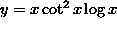.

• PROBLEM 18 : Consider the function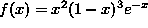. For what values of x is f'(x) = 0 ?

• PROBLEM 19 : Find an equation of the line tangent to the graph ofat.

• PROBLEM 20 : Find an equation of the line perpendicular to the graph ofat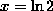.

• PROBLEM 21 : Find all points (x, y) on the graph ofwith tangent lines parallel to the line y + x = 12 .